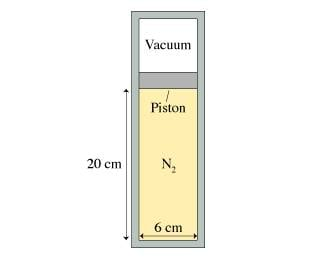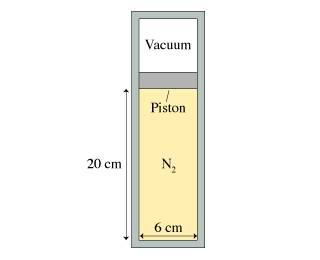# Im having trouble with a simple question (simple thermodynamics)

pureouchies4717
HI! i was wondering if someone could please help me with this physics problem. it looks very simple, and I am only missing one value. thanks

A 6.0-cm-diameter cylinder of nitrogen gas has a 4.0-cm-thick movable copper piston. The cylinder is oriented vertically, as shown in the figure, and the air above the piston is evacuated. When the gas temperature is 20 degree C, the piston floats 20 cm above the bottom of the cylinder.What is the gas pressure?gas pressure is: p= F/A = (mg)/A(of the cylinder)

my problem is: how can i get the mass of the piston? its really annoying me. please help. thanks

Homework Helper
nick727kcin said:
HI! i was wondering if someone could please help me with this physics problem. it looks very simple, and I am only missing one value. thanksgas pressure is: p= F/A = (mg)/A(of the cylinder)

my problem is: how can i get the mass of the piston? its really annoying me. please help. thanks
What is the volume and density of the piston? (ie. what is the density of copper?).

AM

pureouchies4717
thanks for responding!

the density is: 8.96 g/cm^3

the volume of the piston is: [(3.14) x (.03)^2].04 = .000113 m^3 = 113.0973 cm^3

so then, i need to multiply : M= 113.0973 x 8.96 = 1013.35212g

The area of the container is: A= [(3.14 x .03^2)] = .002827

So then: p = Mg/A = 3512858.979

this is wrong though... can you plesae help me find what i did wrong :grumpy:

Last edited:
Homework Helper
nick727kcin said:
thanks for responding!

the density is: 8.96 g/cm^3

the volume of the piston is: [(3.14) x (.03)^2].04 = .000113 m^3 = 113.0973 cm^3

so then, i need to multiply : M= 113.0973 x 8.96 = 1013.35212g

The area of the container is: A= [(3.14 x .03^2)] = .002827

So then: p = Mg/A = 3512858.979

this is wrong though... can you plesae help me find what i did wrong :grumpy:
First of all, work out the solution algebraically and then plug in numbers. Second, you should stick to significant figures.

Mass of piston = $\rho V = \rho\pi r^2h$
Pressure = Mg/A = $\rho\pi r^2hg/\pi r^2 = \rho hg$

g = 9.80 m/sec^2
$\rho = 8.96 g/cm^3 = 8.96 x 10^-3 kg/10^{-6} m^3=8.96 x 10^3 kg/m^3$

Pressure = 8960 x .04 (9.80) = 3512 N/m^2 = 3512 Pa. = 3.512 kpa

In significant figures, this would be 3.5 kpa

AM